•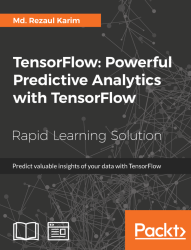#### TensorFlow: Powerful Predictive Analytics with TensorFlow#### Overview of this book

Predictive analytics discovers hidden patterns from structured and unstructured data for automated decision making in business intelligence. Predictive decisions are becoming a huge trend worldwide, catering to wide industry sectors by predicting which decisions are more likely to give maximum results. TensorFlow, Google’s brainchild, is immensely popular and extensively used for predictive analysis. This book is a quick learning guide on all the three types of machine learning, that is, supervised, unsupervised, and reinforcement learning with TensorFlow. This book will teach you predictive analytics for high-dimensional and sequence data. In particular, you will learn the linear regression model for regression analysis. You will also learn how to use regression for predicting continuous values. You will learn supervised learning algorithms for predictive analytics. You will explore unsupervised learning and clustering using K-meansYou will then learn how to predict neighborhoods using K-means, and then, see another example of clustering audio clips based on their audio features. This book is ideal for developers, data analysts, machine learning practitioners, and deep learning enthusiasts who want to build powerful, robust, and accurate predictive models with the power of TensorFlow. This book is embedded with useful assessments that will help you revise the concepts you have learned in this book. This book is repurposed for this specific learning experience from material from Packt's Predictive Analytics with TensorFlow by Md. Rezaul Karim.
TensorFlow: Powerful Predictive Analytics with TensorFlowCreditsPrefaceFree Chapter
From Data to Decisions – Getting Started with TensorFlowPutting Data in Place – Supervised Learning for Predictive AnalyticsClustering Your Data – Unsupervised Learning for Predictive AnalyticsUsing Reinforcement Learning for Predictive Analytics## Taking Decisions Based on Data – Titanic Example

The growing demand for data is a key challenge. Decision support teams such as institutional research and business intelligence often cannot take the right decisions on how to expand their business and research outcomes from a huge collection of data. Although data plays an important role in driving the decision, however, in reality, taking the right decision at right time is the goal.

In other words, the goal is the decision support, not the data support. This can be achieved through an advanced use of data management and analytics.

### Data Value Chain for Making Decisions

The following diagram in figure 1 (source: H. Gilbert Miller and Peter Mork, From Data to Decisions: A Value Chain for Big Data, Proc. Of IT Professional, Volume: 15, Issue: 1, Jan.-Feb. 2013, DOI: 10.1109/MITP.2013.11) shows the data chain towards taking actual decisions–that is, the goal. The value chains start through the data discovery stage consisting of several steps such as data collection and annotating data preparation, and then organizing them in a logical order having the desired flow. Then comes the data integration for establishing a common data representation of the data. Since the target is to take the right decision, for future reference having the appropriate provenance of the data–that is, where it comes from, is important: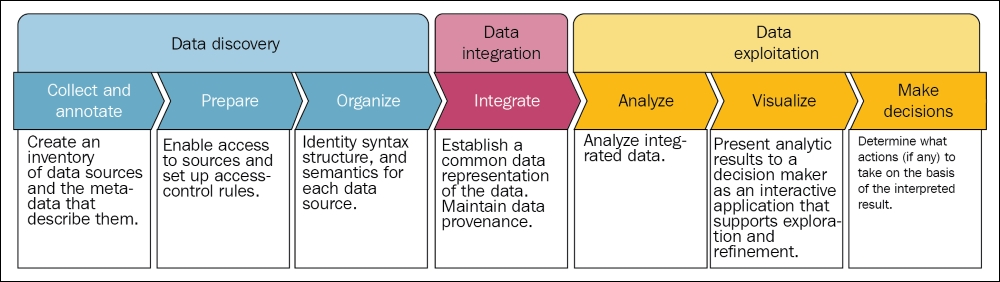Figure 1: From data to decisions: a value chain for big data

Well, now your data is somehow integrated into a presentable format, it's time for the data exploration stage, which consists of several steps such as analyzing the integrated data and visualization before taking the actions to take on the basis of the interpreted results.

However, is this enough before taking the right decision? Probably not! The reason is that it lacks enough analytics, which eventually helps to take the decision with an actionable insight. Predictive analytics comes in here to fill the gap between. Now let's see an example of how in the following section.

### From Disaster to Decision – Titanic Survival Example

Here is the challenge, Titanic–Machine Learning from Disaster from Kaggle (https://www.kaggle.com/c/titanic):

"The sinking of the RMS Titanic is one of the most infamous shipwrecks in history. On April 15, 1912, during her maiden voyage, the Titanic sank after colliding with an iceberg, killing 1502 out of 2224 passengers and crew. This sensational tragedy shocked the international community and led to better safety regulations for ships. One of the reasons that the shipwreck led to such loss of life was that there were not enough lifeboats for the passengers and crew. Although there was some element of luck involved in surviving the sinking, some groups of people were more likely to survive than others, such as women, children, and the upper-class. In this challenge, we ask you to complete the analysis of what sorts of people were likely to survive. In particular, we ask you to apply the tools of machine learning to predict which passengers survived the tragedy."

But going into this deeper, we need to know about the data of passengers travelling in the Titanic during the disaster so that we can develop a predictive model that can be used for survival analysis.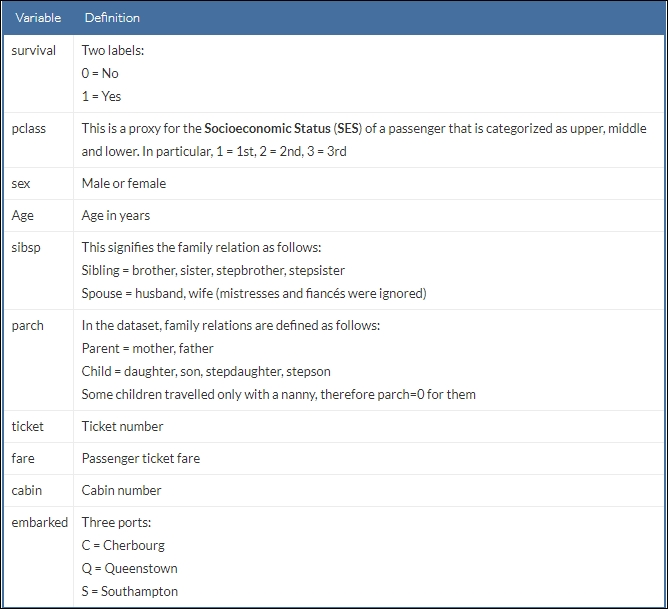A snapshot of the dataset can be seen as follows: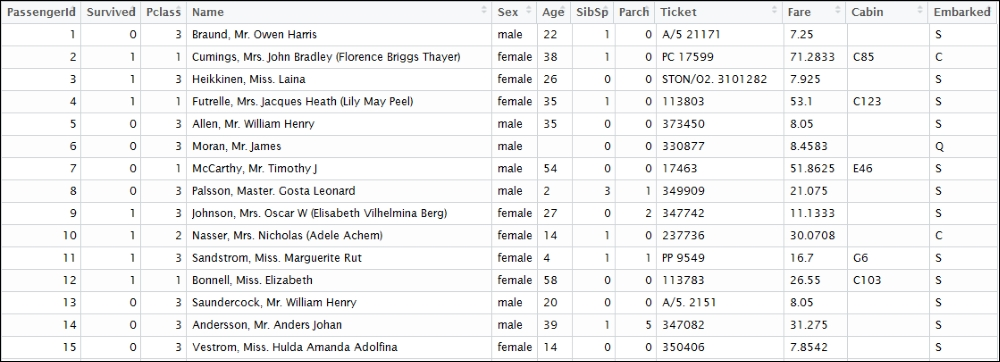Figure 2: A snapshot of the Titanic survival dataset

The ultimate target of using this dataset is to predict what kind of people survived the Titanic disaster. However, a bit of exploratory analysis of the dataset is a mandate. At first, we need to import necessary packages and libraries:

```import pandas as pd
import matplotlib.pyplot as plt
import numpy as np```

Now read the dataset and create a panda's DataFrame:

`df = pd.read_csv('/home/asif/titanic_data.csv')`

Before drawing the distribution of the dataset, let's specify the parameters for the graph:

```fig = plt.figure(figsize=(18,6), dpi=1600)
alpha=alpha_scatterplot = 0.2
alpha_bar_chart = 0.55
fig = plt.figure()

Draw a bar diagram for showing who survived versus who did not:

```ax1 = plt.subplot2grid((2,3),(0,0))
ax1.set_xlim(-1, 2)
df.Survived.value_counts().plot(kind='bar', alpha=alpha_bar_chart)
plt.title("Survival distribution: 1 = survived")```

Plot a graph showing survival by `Age`:

```plt.subplot2grid((2,3),(0,1))
plt.scatter(df.Survived, df.Age, alpha=alpha_scatterplot)
plt.ylabel("Age")
plt.grid(b=True, which='major', axis='y')
plt.title("Survival by Age: 1 = survived")```

Plot a graph showing distribution of the `passengers` classes:

```ax3 = plt.subplot2grid((2,3),(0,2))
df.Pclass.value_counts().plot(kind="barh", alpha=alpha_bar_chart)
ax3.set_ylim(-1, len(df.Pclass.value_counts()))
plt.title("Class dist. of the passengers")```

Plot a kernel density estimate of the subset of the 1st class passengers' age:

```plt.subplot2grid((2,3),(1,0), colspan=2)
df.Age[df.Pclass == 1].plot(kind='kde')
df.Age[df.Pclass == 2].plot(kind='kde')
df.Age[df.Pclass == 3].plot(kind='kde')
plt.xlabel("Age")
plt.title("Age dist. within class")
plt.legend(('1st Class', '2nd Class','3rd Class'),loc='best')```

Plot a graph showing `passengers per boarding location`:

```ax5 = plt.subplot2grid((2,3),(1,2))
df.Embarked.value_counts().plot(kind='bar', alpha=alpha_bar_chart)
ax5.set_xlim(-1, len(df.Embarked.value_counts()))
plt.title("Passengers per boarding location")
Finally, we show all the subplots together:
plt.show()
>>>```

The figure shows the survival distribution, survival by age, age distribution, and the passengers per boarding location: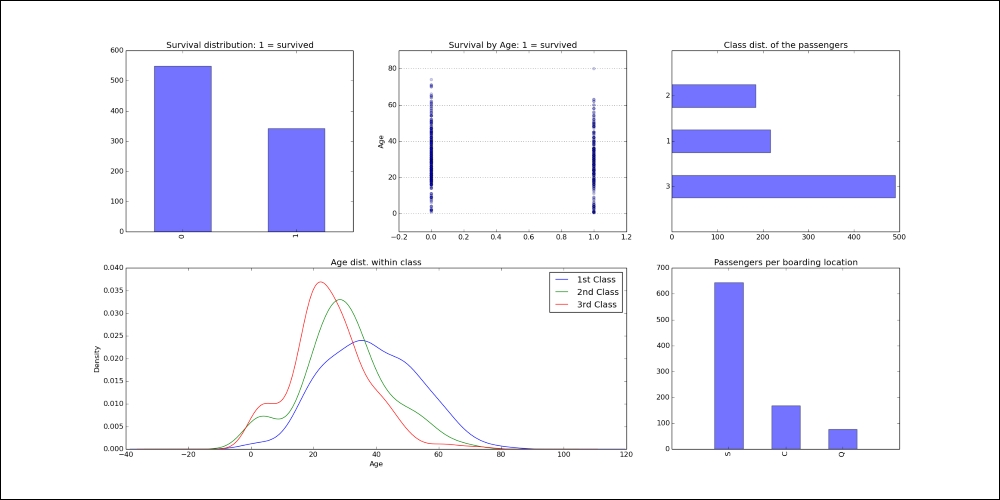Figure 3: Titanic survival data distribution across age, class, and age within classes and boarding location

However, to execute the preceding code, you need to install several packages such as matplotlib, pandas, and scipy. They are listed as follows:

• Installing pandas: Pandas is a Python package for data manipulation. It can be installed as follows:

```\$ sudo pip3 install pandas
#For Python 2.7, use the following:
\$ sudo pip install pandas
```
• Installing matplotlib: In the preceding code, matplotlib is a plotting library for mathematical objects. It can be installed as follows:

```\$ sudo apt-get install python-matplotlib   # for Python 2.7
\$ sudo apt-get install python3-matplotlib # for Python 3.x
```
• Installing scipy: Scipy is a Python package for scientific computing. Installing `blas` and `lapack`and `gfortran` are a prerequisite for this one. Now just execute the following command on your terminal:

```\$ sudo apt-get install libblas-dev liblapack-dev \$ sudo apt-get install gfortran \$ sudo pip3 install scipy # for Python 3.x
\$ sudo pip install scipy # for Python 2.7
```

For Mac, use the following command to install the above modules:

```\$ sudo easy_install pip
\$ sudo pip install matplotlib
\$ sudo pip install libblas-dev liblapack-dev
\$ sudo pip install gfortran
\$ sudo pip install scipy
```

For windows, I am assuming that Python 2.7 is already installed at C:\Python27. Then open the command prompt and type the following command:

```C:\Users\admin-karim>cd C:/Python27
C:\Python27> python -m pip install <package_name> # provide package name accordingly.
```

For Python3, issue the following commands:

```C:\Users\admin-karim>cd C:\Users\admin-karim\AppData\Local\Programs\Python\Python35\Scripts